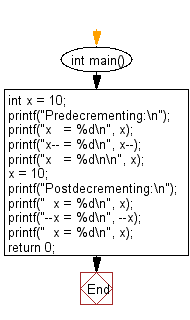﻿ C Program: Compares predecrementing with postdecrementing

# C Exercises: Demonstrates the difference between predecrementing and postdecrementing using the decrement operator

## C Basic Declarations and Expressions: Exercise-78 with Solution

Write a C program to demonstrate the difference between predecrementing and postdecrementing using the decrement operator --.

Sample Solution:

C Code:

``````#include<stdio.h>
int main()
{
int x = 10; // Initialize variable x with value 10

// Predecrementing
printf("Predecrementing:\n");
printf("x   = %d\n", x); // Print current value of x
printf("x-- = %d\n", x--); // Print x and then decrement it
printf("x   = %d\n\n", x); // Print updated value of x

x = 10; // Reset x to 10

// Postdecrementing
printf("Postdecrementing:\n");
printf("  x = %d\n", x); // Print current value of x
printf("--x = %d\n", --x); // Decrement x and then print it
printf("  x = %d\n", x); // Print updated value of x

return 0;
}
``````

Sample Output:

```Predecrementing:
x   = 10
x-- = 10
x   = 9
```

Flowchart:C programming Code Editor:

What is the difficulty level of this exercise?

Test your Programming skills with w3resource's quiz.

﻿